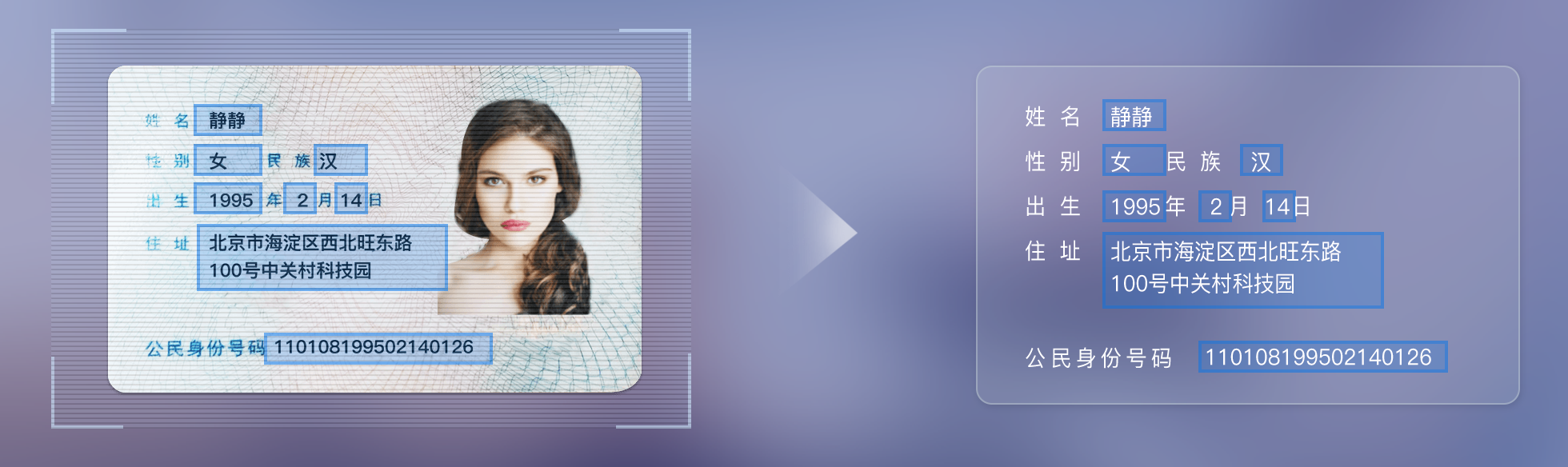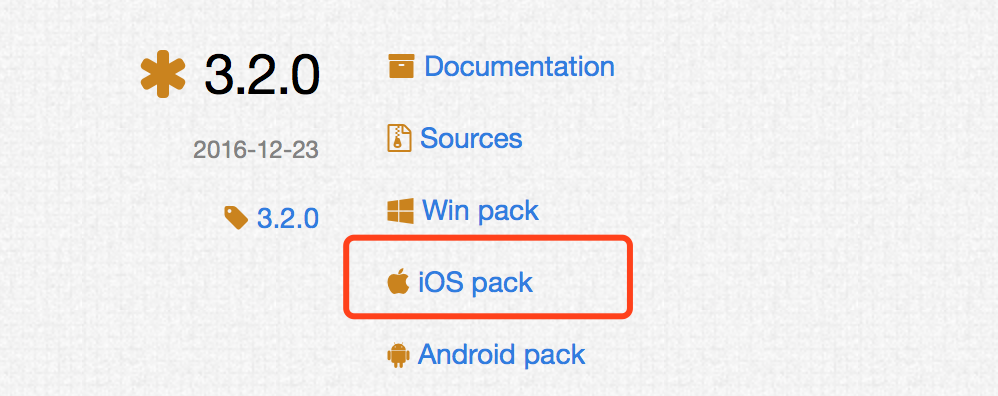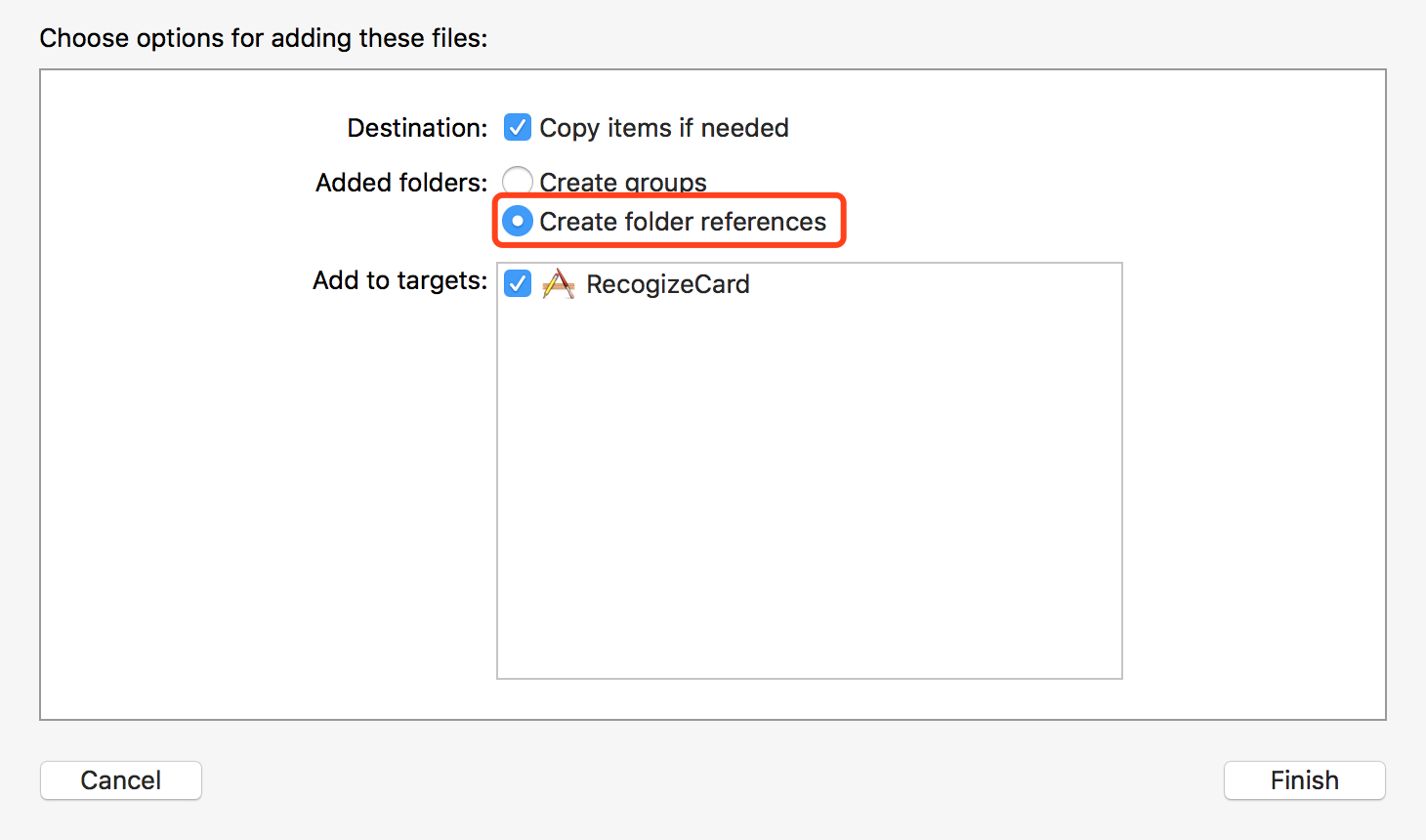# 曾静的博客

`OCR``Optical Character Recognition`，光学字符识别）是指电子设备（例如扫描仪或数码相机）检查纸上打印的字符，通过检测暗、亮的模式确定其形状，然后用字符识别方法将形状翻译成计算机文字的过程。通俗来说就是通过对图像进行处理提取裁剪出来有字符的区域然后对字符进行识别翻译成文字。### 环境准备

#### 1、配置Cocoapods Podfile

``````pod 'OpenCV', '~> 3.2.0'
pod 'TesseractOCRiOS', '~> 4.0.0'
``````#### 2、下载tesseract的训练库

``````git clone https://github.com/tesseract-ocr/tessdata.git
``````

``````git checkout 3.04.00
``````### 操作步骤

#### 1、图像处理

##### (1) 转化为灰度图像
``````cv::cvtColor(src, dest, cv::COLOR_BGR2GRAY);
``````
##### (2) 二值化
``````cv::threshold(src, dest, 100, 255, CV_THRESH_BINARY);
``````
##### (3) 图像腐蚀填充

``````cv::Mat erodeElement = getStructuringElement(cv::MORPH_RECT, cv::Size(26, 26));
cv::erode(src, dest, erodeElement);
``````
##### (4) 轮廓检测

``````CV_EXPORTS void findContours(InputOutputArray image, OutputArrayOfArrays contours, int mode, int method, Point offset = Point());
``````

• image ：要寻找轮廓的图片，注意这里的轮廓会直接改变在src上(需要备份)；
• contours：输出检测到的轮廓
• mode：轮廓检索模式。CV_RETR_TREE：检索所有的轮廓
• method: 轮廓近似方法。CV_CHAIN_APPROX_SIMPLE ：表示去掉冗余信息
• offset: 搜索的偏移

``````std::vector<std::vector<cv::Point>> contours;//定义一个容器来存储所有检测到的轮廊
cv::findContours(src, contours, CV_RETR_TREE, CV_CHAIN_APPROX_SIMPLE, cvPoint(0, 0));
``````
##### (5) 身份证号码提取

``````std::vector<cv::Rect> rects;
cv::Rect numberRect = cv::Rect(0,0,0,0);
std::vector<std::vector<cv::Point>>::const_iterator itContours = contours.begin();
for ( ; itContours != contours.end(); ++itContours) {
cv::Rect rect = cv::boundingRect(*itContours);
rects.push_back(rect);
//算法原理: 宽度/高度 > 5
if (rect.width > numberRect.width && rect.width > rect.height * 5) {
numberRect = rect;
}
}
``````

#### 2、信息提取

``````- (void)pd_recognizeImageWithTesseract:(UIImage *)image complete:(void (^)(BOOL status, NSString *result))complete {
dispatch_async(dispatch_get_global_queue(DISPATCH_QUEUE_PRIORITY_BACKGROUND, 0), ^{
G8Tesseract *tesseract = [[G8Tesseract alloc] initWithLanguage:@"eng"];
tesseract.image = [image g8_blackAndWhite];
tesseract.charWhitelist = @"0123456789";
BOOL status = [tesseract recognize];
dispatch_async(dispatch_get_main_queue(), ^{
complete(status, tesseract.recognizedText);
});
});
}
``````

#### 3、说明：

• （1）测试素材可以从`https://cloud.baidu.com/product/ocr/idcard`获取
• （2）优化的方向有几个方面：
• ① 调整提取号码区域的算法（腐蚀与填充、号码区域提取）
• ② 手动进行样本训练

Note继续阅读

Java继续阅读